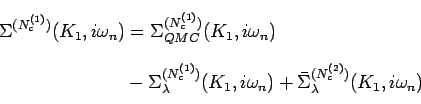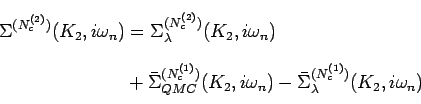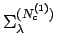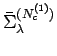Next: 3.3 Approximations to the Up: 3.2 Formalism Previous: 3.2.3 A Conserving Approximation

## 3.2.4 Ansatz

To account for the omitted set of short length-scale self-energy diagrams in the-approximation, we substitute appropriate diagrams from the small cluster QMC result. This diagrammatic substitution between the different length-scales in the MSMB method is done by means of an analytic Ansatz.

One possible implementation of the MSMB method which yields a self-energy containing correlations on both long and short length-scales is given by the real-space Ansatz:(3.10)

In this formalism, the MSMB self-energy is constructed by taking all contributions of lengths up to the linear sizes of the small cluster () from the exact QMC result and the remaining longer ranged contributions are complemented by the-approximated large cluster self-energy. Note, the multi-scale self-energy obtained in Eq. 3.10 has lost its spatial continuity. Fourier-transforming the individual length-scale contributions in Eq. 3.10 yields(3.11)

where only diagonal parts of the Fourier transformed self-energy are considered.

J. Hague et. al. in ref. (3) considered the following, alternative momentum-space Ansatz to combine the different length-scales:(3.12)(3.13)

whereis the self-energy obtained in the-approximation when implemented on the small cluster. The self-energiesandon the small and large cluster, respectively, exist on different grid sizes, and it becomes necessary to convert self-energies from one to the other. This conversion is denoted by a bar over the self-energy which denotes an interpolation when going from a coarser grid to a finer one and a coarse-graining step otherwise. For example, the large cluster self-energyis constructed from the explicit-approximated self-energy on the large cluster, and two interpolated small cluster (denoted by the superscript) self-energiesand. It is important to note that the coarse graining in going from large- to small-cluster self-energies is not an averaging over electron propagators but the term is used in this context to imply an averaging of the self-energy within a cluster-cell.

While the real space implementation of the Ansatz was a straight forward combination of different length-scale elements, the momentum implementation interpretation is more involved. The-method provides an estimate for the set of self-energy diagrams which convey the long-ranged correlations of the system. However, since in reciprocal space there isn't an explicit separation of length-scales, the remaining short length-scale diagrams which are to be supplied by the QMC calculation have to be identified. This can be accomplished by removing the sub-set of-approximated self-energy diagrams on the small cluster from the complete set of the QMC calculation hence avoiding a double counting of the corresponding self-energy contributions.

Within the traditional form of the DCA, all self-energies are inherently causal for each individual cluster. One consequence of causality is that. In this Ansatz based implementation of the MSMB method however, causality is not inherently guaranteed. The combination of self-energy contributions of various length-scales in the Ansatz is only assured to yield a causal multi-scale self-energy in the limit of the cluster sizes approaching one another. Therefore, causality in these schemes cannot be guaranteed and hence has to be monitored closely throughout. For a more detailed discussion of this and the entire momentum-space Ansatz see Ref. (3).

The remaining self-consistent implementation of the multi scale method (i.e. two cluster DCA) is similar to that of the traditional, single cluster DCA. Fig. 3.5 depicts a flow chart of the implementation of both Ansätze in the scheme of the overall self-consistency loop. The bottom loop shows the already discussed DCA self-consistency loop using the QMC as a small cluster solver. For the large cluster solver (-method) the self-consistency is similar. In the overall scheme of the Ansatz, the two cluster problems are combined in a fully self-consistent approach: After each iteration of the QMC/DCA loop, the corresponding/DCA contribution on the large cluster is evaluated. The Ansatz is used after each step to calculate new estimates for the self-energies on both the small and large cluster, thus yielding a fully self-consistent solution. The corresponding paths are shown in Fig. 3.5. The converged Ansatz self-energy will be dominated by the small cluster QMC self-energy which contributes the strongest, short-ranged correlations. The remaining weaker long-ranged correlations are incorporated in the difference of-approximated self-energies between the two clusters. In moving away from a fully self-consistent approach, the self-consistency restrictions may be lessened to various degrees. Some possible implementations will be discussed in further detail in section 3.4.Next: 3.3 Approximations to the Up: 3.2 Formalism Previous: 3.2.3 A Conserving Approximation
© Cyrill Slezak